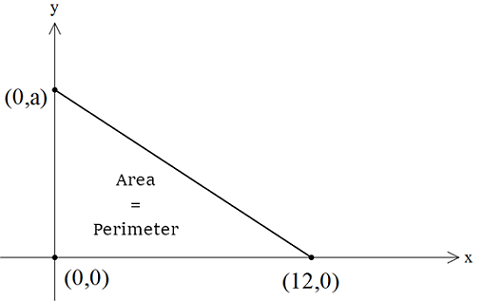Geometry Level 1A non-degenerate right-angled triangle has vertices at $(0, 0), (12, 0)$ and $(0, a)$ with $a>0$. Its perimeter is numerically equal to its area. What is $a$?

×